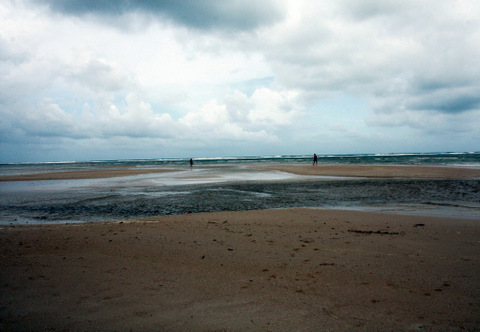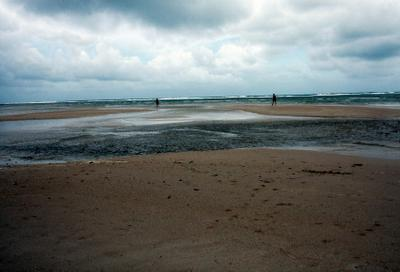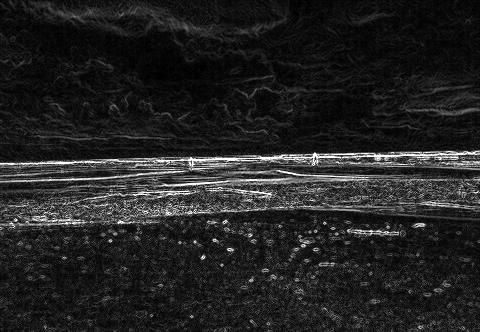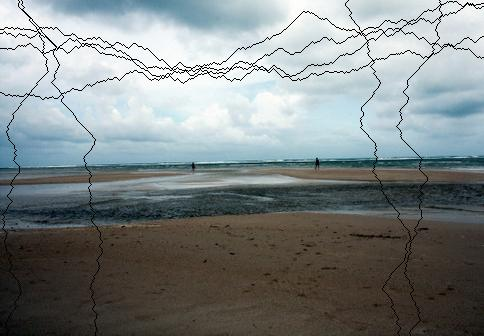# Seam Carving Algorithm

## Intro

Seam carving is an algorithm for content-aware image resizing, it was described in the paper by S. Avidan & A. Shamir. In contract to stretching, content-aware resizing allows to remove/add pixels which has less meaning while saving more important. Pictures below demonstrate this - original picture of the size 332x480 is on the top, the picture after applying seam carving (size is 272x400) is on the bottomThis algorithm is quite impressive so one may find a lot of articles describing it. Yet as I found most of the authors haven’t read the original paper and provide a very basic implementation. In this post I will describe the algorithm with all details as it was written by Avidan & Shamir. Yet I will write from the programmers point of view, without going into Math too deep. In addition to algorithms description, I also provide Matlab code.

## Energy

For simplification, we will describe only reducing the size of the image. But enlarging process is very similar and described in the last section. The idea is to remove content that has smaller meaning for the user (contain less information). We will call this information “energy”. Thus we need to introduce an energy function that would map a pixel into energy value. For instance, we can use gradient of the pixel: . If a picture has 3 channels, just sum values of the energy in each channel. The Matlab code below demostates it. The imfilter function just applies the mask to each pixel, so the result dI(i, j)/dx = I(i+1)-I(i-1)/dx where dx = 1.

Here is energy function:## Seam

If we delete pixels with minimum energy but random positions, we will get distorted picture. If we delete columns/rows with minimum energy, we will get artifacts. Here by column I mean {(i, j)| j is predefined}, row - {(i, j)| i is predefined}. The solution is to introduce a generalization of column/row (called seam). Formally, let I is n x m image, then a vertical seam is , where x: [1,..,n] -> [1,..,m]. It means that a vertical seam is path from the top of the picture to the bottom such that the length of the path in pixels is width of the image, and for each seam element (i,j), the next seam element can be only (i+1, j-1), (i+1, j), (i+1, j+1). Similarly, we can define a horizontal seam. Examples of seams are shown on the figure below in black:We are looking for a seam with the minimum energy among all seams (in chosen dimension): . The way to find such an optimal way is by using dynamic programming:

1. Find M - minimum energy for all possible seams for each (i, j):
• fill in the first row by energy
• for all rows starting from second: M[i, j] = e[i, j] + min(M[i - 1, j], M[i, j], M[i + 1, j]);
2. Find the minimum value in the last row of M and traverse back choosing pixels with minimum energy.

Note that in Matlab code I had to represent seam as n x m bit matrix. If pixel is in the seam it is 0, otherwise 1.

In order to find a horizontal seam, just pass a transposed energy matrix to findOptSeam.

## Find optimal order of deleting seams

Now we can compute seams and using the code below we can even remove them from an image:

It is already a good tool for reducing image in one dimension - just find and delete seam as many times as you need. But what if you need to reduce the size of the image in both directions? How to decide at every iteration whether it is better (in terms of energy minimization) to delete a column or a row? This problem is solved, again, using dynamic programming. Let n’ x m’ are desirable size of the image (n’ < n, m’ < m). We introduce a transport matrix T which defines for every n’ x m’ the cost of the optimal sequence of horizontal and vertical seam removal operations. It is more suitable to introduce r = n - n’ and c = m - m’ which defines number of horizontal and vertical removal operations. In addition to T we introduce a map of the size r x c TBM which specifies for every T(i, j) whether we came to this point using horizontal (0) or vertical (1) seam removal operation. Pseudocode is shown below:

And Matlab imeplementation:

## Enlarging an image

In order to enlarge a picture, we compute k optimal seams for deleting but then, instead of deleting, copy average between neighbors:

## Source code

The full code of the program is available here. Seam carving is also implemented in ImageMagick. So if you need a C++ implementation, check out ImageMagick code.